Private GMAT Tutoring in NYC & Online

# GMAT Question of the Day - Data Sufficiency - Absolute Value

Is x > 0?

(1) 3x - 2 = |3-x|

(2) x^2 > 1

## GMAT Question of the Day Solution

Most GMAT tutoring students don't appreciate absolute value questions. Especially in the Data Sufficiency. That's understandable because GMAT absolute value questions can be tricky, especially if you don't approach them in an organized fashion. So how should you think about them? Whenever you see an absolute value question think about the signs (positive/negative). Think about what happens when the number in the absolute is positive and what happens when the number is negative. Also remember that an absolute value can't be negative. In a general sense that's all you have to worry about.

In this case we want to know if x is positive. If we're thinking in positive and negative we should see that the right side of the equation must be greater than or equal to zero (because it is an absolute value). So you can re-write the equation 3x - 2 is greater than or equal to 0. Solve and you will see that x must be positive. Sufficient. Statement (2) doesn't help us decide. You can pick and positive/negative value for x. This statement is just there for someone who doesn't understand the question and so might consider going for a seemingly safe "c" choice. Insufficient.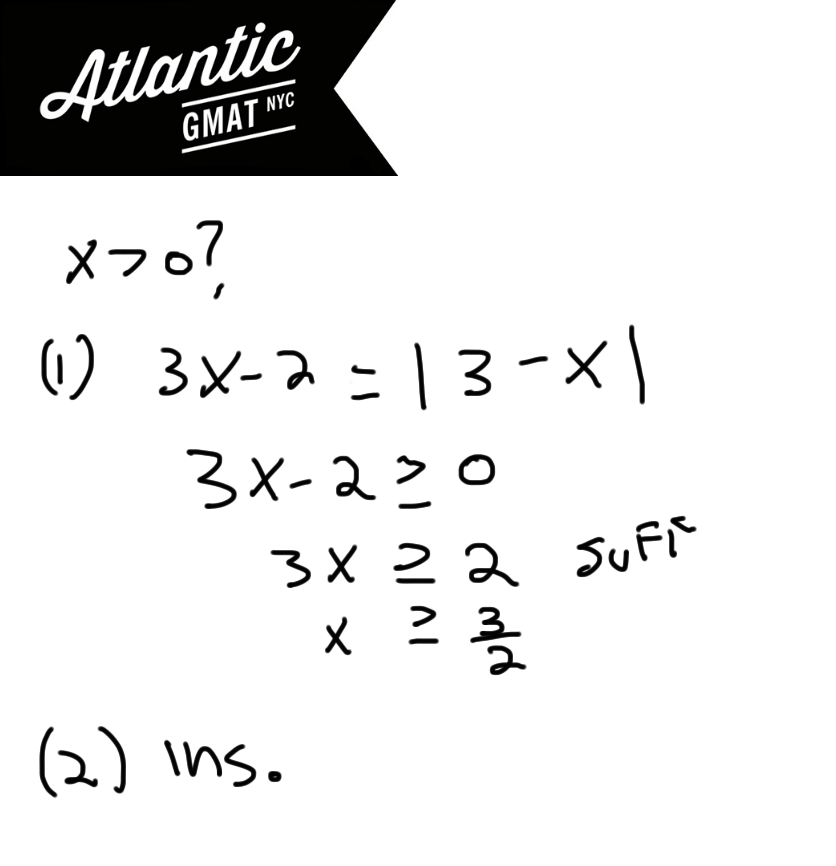# GMAT Question of the Day - Data Sufficiency - Number Properties

How many integers between x and 10! are divisible by 3?

(1) x^2 = x

(2) -x|x| = x

<

## GMAT Question of the Day Solution

This GMAT question of the day presents what seems like a giant universe of options. When you see a GMAT question similar to this you don't need to worry because usually the universe is much smaller than it seems. Let's just stay focused on the question. In this we're not solving for a specific value. We just need to know about the number of numbers that are divisible by three between x and 10!. Statement (1) tells us that x is either 1 or 0. You might say insufficient because there are two possibilities for x but remember that we are not solving for x. x = 1 and x = 0 produce the same outcome because the first integer that is divisible by three is three itself. Of course 0 is divisible by three (and every other number) but zero doesn't count because the questions use the word "between" which means non inclusive. Sufficient. Statement (2) tells us that x must be 0. Sufficient.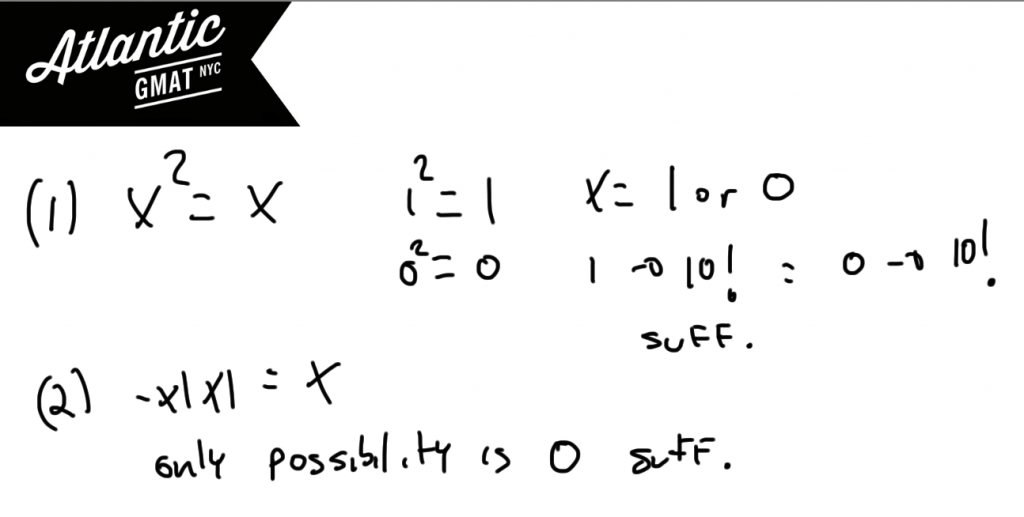# GMAT Question of the Day - Data Sufficiency - Absolute Value

If |x| is greater than or equal to 1 is x < 0?

(1) 1/2 - |x|/x > 0

(2) |x|/2 - x/3 > 0

# GMAT Question of the Day Solution

For GMAT Absolute value questions remember to think about what happens when the number is negative and what happens when the number is positive. This framework should help you focus on the right information.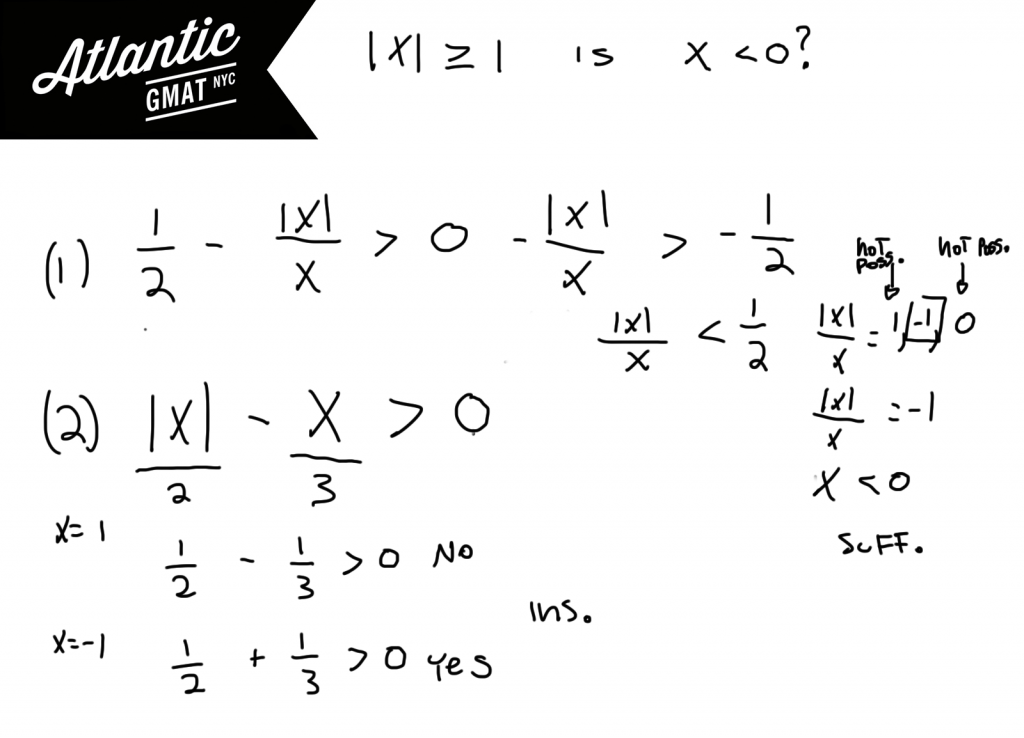# GMAT Question of the Day - Data Sufficiency - Absolute Value/Algebra

x and y are integers. If x > |y| is x > 2?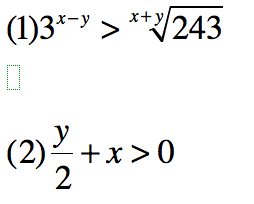## GMAT Question of the Day Solution

GMAT absolute value questions generally focus on the polarity of the variables. Think of what happens when things are positive and when things are negative. In this case we know that x will be positive because of the given information. Now we have to decide whether x will be greater than 2. The first statement proves that x will in fact be greater than two. The second leaves open many possibilities. If you struggled with the exponents/roots in the first statement it's time to review your exponent rules.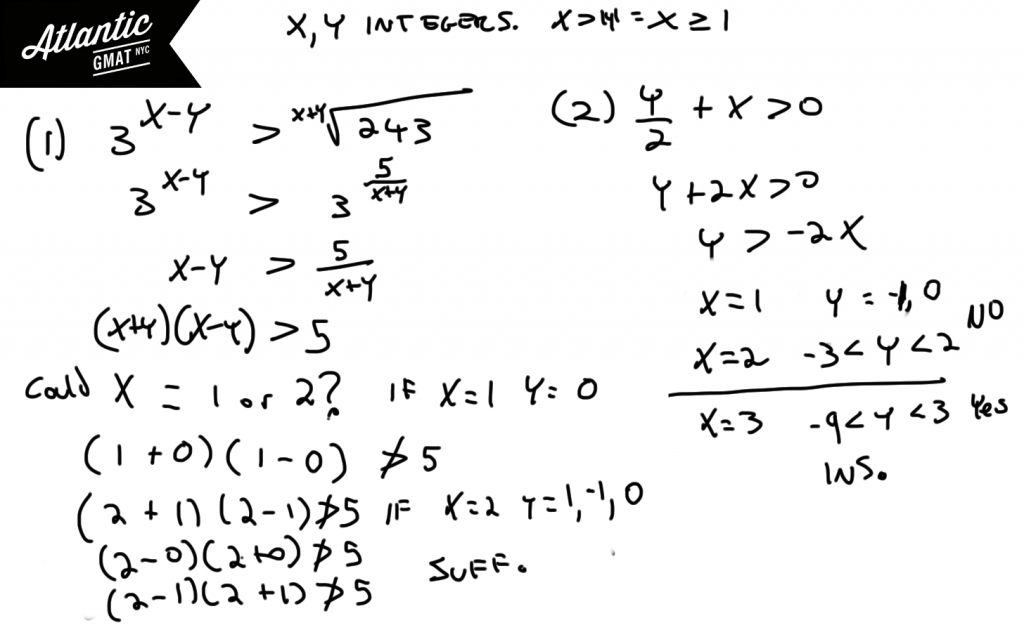# GMAT Question of the Day - Data Sufficiency - Absolute Value

If x is an integer is y < 2?

(1) |x| - x = y

(2) x < |x|

## GMAT Question of the Day Solution

For GMAT absolute value questions think about the signs (+, -). What happens when the underlying variable is positive? What happens when it is negative? In statement (1) we can see that a positive value of x will yield a value of zero for y whereas a negative value for x will yield a value of -2x for y. This leaves us with many possible values and so statement (1) is insufficient. Statement (2) doesn't tell us anything about y but tells us that x must be negative. Insufficient. Putting the statements together y must equal -2x and x must be negative. So the least possible value for y is 2 (when x is -1). This means that y cannot be less than 2. Sufficient.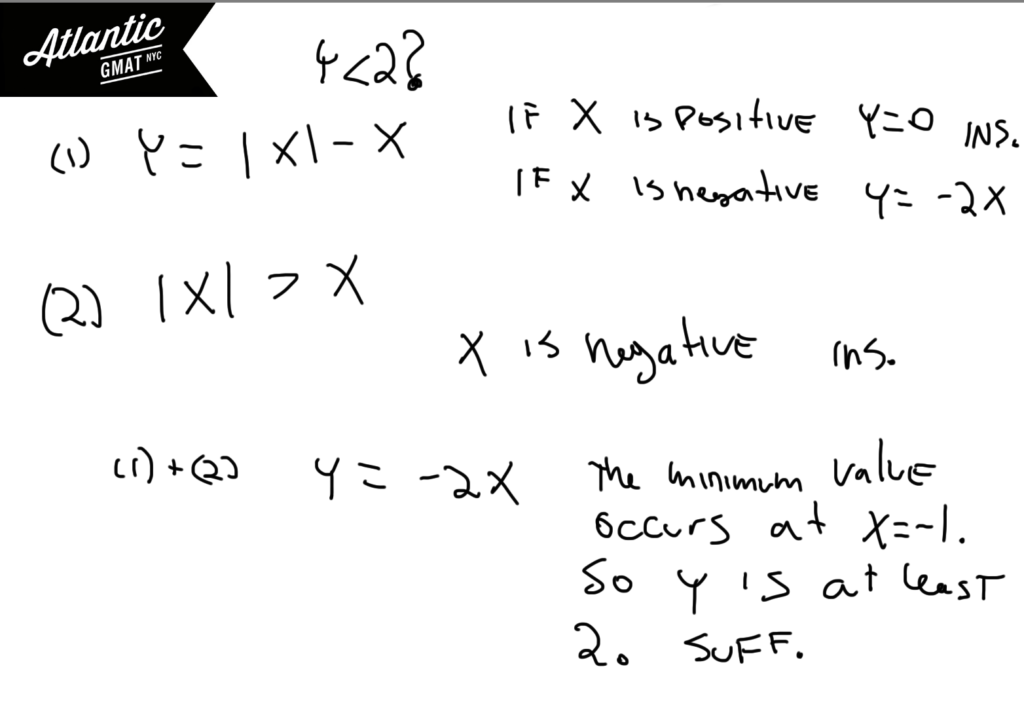# CONTACT

Atlantic GMAT

405 East 51st St.

NY, NY 10022

(347) 669-3545

info@AtlanticGMAT.com